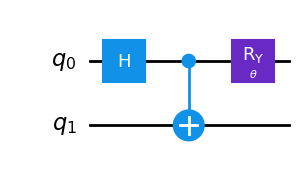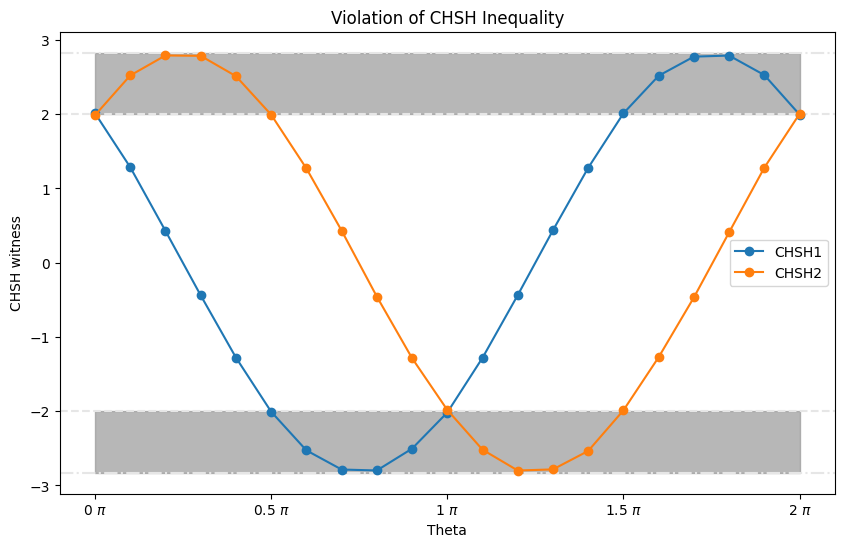Learning Home Catalog Composer Lab
Learning
Home Catalog Composer Lab
Demonstrate the violation of the CHSH inequality with the Estimator primitive
IntroductionBackgroundCHSH inequalityOverviewSetupConnect to the Qiskit Runtime serviceStep 1: Create CHSH circuitsCreate a parameterized CHSH circuitCreate a list of phase values to be assigned laterObservablesStep 2: Submit the circuits to a quantum computer on the cloudStep 3: Analyze the resultsSummary

# Demonstrate the violation of the CHSH inequality with the Estimator primitive

## Introduction

In this tutorial, you will run an experiment on a quantum computer to demonstrate the violation of the CHSH inequality with the Estimator primitive.

The violation of the CHSH inequality is used to show that quantum mechanics is incompatible with local hidden-variable theories. This is an important experiment for understanding the foundation of quantum mechanics. The 2022 Nobel Prize for Physics was awarded to Alain Aspect, John Clauser and Anton Zeilinger in part for their pioneering work in quantum information science, and in particular, their experiments with entangled photons demonstrating violation of Bell’s inequalities. An experimental method by which one can test Bell’s inequality was put forth by Clauser, Horne, Shimony, and Holt (CHSH) in 1969, and forms the basis for the experiment conducted here. It's truly incredible that you can use Qiskit and an IBM Quantum system to conduct this experiment from the comfort of your home!

## Background

### CHSH inequality

The CHSH inequality, named after the authors Clauser, Horne, Shimony, and Holt, is used to experimentally prove Bell's theorem. This theorem asserts that local hidden variable theories cannot account for some consequences of entanglement in quantum mechanics.

For this experiment, we will create an entangled pair on which we measure each qubit on two different bases. We will label the bases for the first qubit $A$ and $a$ and the bases for the second qubit $B$ and $b$. This allows us to compute the CHSH quantity $S_1$:

$S_1 = A(B-b) + a(B+b).$

Each observable is either $+1$ or $-1$. Clearly, one of the terms $B\pm b$ must be $0$, and the other must be $\pm 2$. Therefore, $S_1 = \pm 2$. The average value of $S_1$ must satisfy the inequality:

$|\langle S_1 \rangle|\leq 2.$

Expanding $S_1$ in terms of $A$, $a$, $B$, and $b$ results in:

$|\langle S_1 \rangle| = |\langle AB \rangle - \langle Ab \rangle + \langle aB \rangle + \langle ab \rangle| \leq 2$

You can define another CHSH quantity $S_2$:

$S_2 = A(B+b) - a(B-b),$

$|\langle S_2 \rangle| = |\langle AB \rangle + \langle Ab \rangle - \langle aB \rangle + \langle ab \rangle| \leq 2$

If quantum mechanics can be described by local hidden variable theories, the previous inequalities must hold true. However, as you are going to demonstrate in this notebook, these inequalities can be violated in a quantum computer. Therefore, quantum mechanics is not compatible with local hidden variable theories.

If you want to learn more, read the section on IBM Quantum Learning or watch John Watrous explain the CHSH Inequality below:

## Overview

You will create an entangled pair between two qubits in a quantum computer by creating the Bell state $|\Phi^+\rangle = \frac{|00\rangle + |11\rangle}{\sqrt{2}}$. Using the Estimator primitive, you can directly obtain the expectation values needed ($\langle AB \rangle, \langle Ab \rangle, \langle aB \rangle$, and $\langle ab \rangle$) to calculate the expectation values of the two CHSH quantities $\langle S_1\rangle$ and $\langle S_2\rangle$. Before the introduction of the Estimator primitive, you would have to construct the expectation values from the measurement outcomes.

You will measure the second qubit in the $Z$ and $X$ bases. The first qubit will be measured also in orthogonal bases, but with an angle with respect to the second qubit, which we are going to sweep between $0$ and $2\pi$. As you will see, the Estimator primitive makes running parameterized circuits very easy. Rather than creating a series of CHSH circuits, you only need to create one CHSH circuit with a parameter specifying the measurement angle and a series of phase values for the parameter.

Finally, you will analyze the results and plot them against the measurement angle. You will see that for certain range of measurement angles, the expectation values of CHSH quantities $|\langle S_1\rangle| > 2$ or $|\langle S_2\rangle| > 2$, which demonstrates the violation of the CHSH inequality.

## Setup

Authenticate to run code cells
Reset Copy to clipboard

Output:

### Connect to the Qiskit Runtime service

Before running circuits, you need to connect to the Qiskit Runtime service instance, and select a target backend:

Authenticate to run code cells
Reset Copy to clipboard

Output:

'ibmq_belem'


## Step 1: Create CHSH circuits

### Create a parameterized CHSH circuit

First, we write the circuit with the parameter $\theta$, which we call theta. The Estimator primitive can enormously simplify circuit building and output analysis by directly providing expectation values of observables. Many problems of interest, especially for near-term applications on noisy systems, can be formulated in terms of expectation values. For maximum generalization, the Estimator primitive requires circuits with no measurements.

Authenticate to run code cells
Reset Copy to clipboard

Output:### Create a list of phase values to be assigned later

After creating the parameterized CHSH circuit, you will create a list of phase values to be assigned to the circuit in the next step. You can use the following code to create a list of 21 phase values range from $0$ to $2 \pi$ with equal spacing, that is, $0$, $0.1 \pi$, $0.2 \pi$, ..., $1.9 \pi$, $2 \pi$.

Authenticate to run code cells
Reset Copy to clipboard

Output:

### Observables

Now we need observables from which to compute the expectation values. In our case we are looking at orthogonal bases for each qubit, letting the parameterized $Y-$ rotation for the first qubit sweep the measurement basis nearly continuously with respect to the second qubit basis. We will therefore choose the observables $ZZ$, $ZX$, $XZ$, and $XX$.

Authenticate to run code cells
Reset Copy to clipboard

Output:

## Step 2: Submit the circuits to a quantum computer on the cloud

In order to execute the entire experiment in one call to the Estimator we need to batch the circuit and operators together, repeating each for the requested number_of_phases points.

Authenticate to run code cells
Reset Copy to clipboard

Output:

We can now create an Estimator instance using our selected backend, and execute our batched circuits and observables:

Authenticate to run code cells
Reset Copy to clipboard

Output:

The individual expectation values can then be extracted from the batched results:

Authenticate to run code cells
Reset Copy to clipboard

Output:

## Step 3: Analyze the results

After running the circuits, we need to build the CHSH witness functions. We first build the quantities $\langle AB \rangle$, $\langle Ab \rangle$, $\langle aB \rangle$, and $\langle ab \rangle$, by looking at the parity of the outcomes for the four families of circuits we built (two measurement bases for each of the two qubits). Then we use those quantities to build the witness functions as defined previously.

Authenticate to run code cells
Reset Copy to clipboard

Output:

Note that now we do not have to build the observables ourselves because they are returned to us! The computed expectation values are given in result.values. What comes back is a very small payload of data.

Authenticate to run code cells
Reset Copy to clipboard

Output:

<matplotlib.legend.Legend at 0x17ce40f70>In the figure, the red dashed lines delimit the classical bounds ($\pm 2$) and the dash-dotted blue lines delimit the quantum bounds ($\pm 2\sqrt{2}$). You can see that the experimental results resemble the general trend of the simulated results and there are regions where the CHSH witness quantities exceeds the classical bounds. Congratulations! You have successfully demonstrated the violation of CHSH inequality in a real quantum system!

Authenticate to run code cells
Reset Copy to clipboard

Output:

## Summary

In this tutorial, you have created a parameterized CHSH circuit, run it using the Estimator primitive, analyzed the results and demonstrated the violation of CHSH inequality. You can see that the Estimator primitive not only makes running parameterized circuits very easy but also greatly simplifies the workflow for obtaining expectation values for observables.

Authenticate to run code cells
Reset Copy to clipboard

Output:

'0.11.3'

Authenticate to run code cells
Reset Copy to clipboard

Output:

'0.25.0'